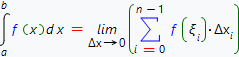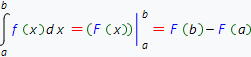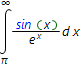# Online definite integrals calculatorDefinite integral of the given function, f(x) is called the limit of integral sums:Definite integral represents the area between the absciss axis, the straight lines x=a, x=b and the given function f(x).

To evaluate definite integral, one should calculate corresponding indefinite integral, and then use Newton-Leibniz integration formula:This formula can only be applied if integrand is continuous at integration interval. So, before start evaluting definite integral, one need to find integrand definition domain. If integrand has some discontinuity points at integration interval, the latter must be splited into intervals where integrand continuous. Then you'd calculate corresponding indefinite integrals on every interval and use Newton-Leibniz formula, to take limits at points where function has discontinuity.

Definite integrals calculatorInput the expression which you want to integrate: